Start typing, then use the up and down arrows to select an option from the list.# Reaction Mechanism Example 3

Jules Bruno
326views
1
1
consider the phone? Elementary steps. What is the rate law of the reaction mechanism? So, if we take a look here, we have a fast step, a slow step and a fast step. So step one is locate the slow step of the reaction mechanism. So here is our slow step. The second elementary step. As long as the reactant are not intermediates, their coefficients will equal the reaction orders of the rate law. So let's let's just focus for right now, on the slow step, we look at the reactant of the slow step and it's one mole of N 02 and one mole of N 205. So for right now we'd say rate equals K N 02 to the one because the coefficient is one and then N 205 Also to the one because the coefficient is one. But if we were to look closer, we'd see that N 02. We have an issue because N 02 is indeed a reactant reaction intermediate because N 02 1st appears as a product and then later as a reactant. Now this leads us to step two. Therefore reacting is an intermediate canceled out with the product intermediate. Alright, so Rno two that we needed from the slow step Gets canceled out by this N here. So that leads us to an issue because if it gets canceled there, that means it gets canceled in our rate law. So now it's no longer there. So this leads us to step three. Now, If step two happens, use the elementary step possessing the product intermediate. Alright, so here, elementary step one. This is our product intermediate. That canceled out with my intermediate from the slope step. What we're gonna do now is gonna take over its reaction. We're going to say for that elementary step, the coefficients, the coefficient coefficients of the reactant still equal the reaction orders. And for that elementary stuff, the coefficients of the products equal the inverse of the reaction orders. So let's see what that means. We come back up here, The N 02 that we needed is now gone. So here we're gonna use the first elementary step we're going to take over. It's reacting its product. We have one mole of N 205 and we have one mole of N 03. If we were to look we see that we have one mole of N here and another mole of N 205 here. This never got canceled out. So we can still use it in total, that's two moles of N 205. So if we come down here Kay, N 205, we have a total of two moles. So reaction orders too. Now the product that we're gonna use Is N 03 and there's one mole of it. So we come down here 1003. Remember we just said that for the elementary step that we're using, the coefficients of the product equals the inverse. So we wouldn't put one year, We put -1 the inverse. So this year would represent our rate law based on the reaction mechanism given to us above. Again, you can see that it gets a bit tricky if one of the reactions that we need from the slow step happens to be an intermediate, because then we have to look at the next elementary step that was involved and we have to incorporate its reactant and its products to give us our overall rate law. Now, this doesn't happen all the time. So in the time that it does just remember these steps that we took in order to find your final rate law.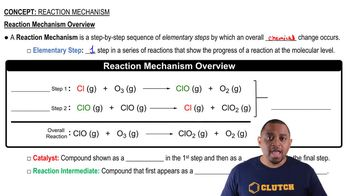03:06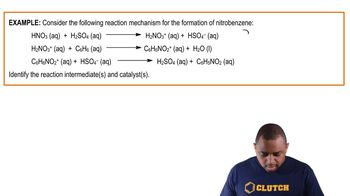02:14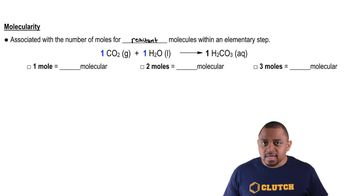01:31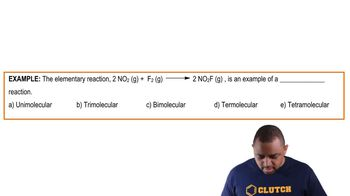01:02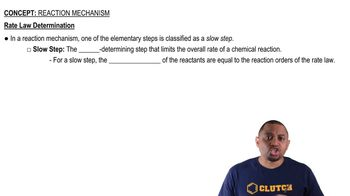00:58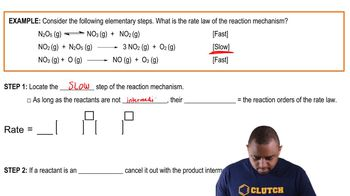04:16